# Volume of 3D Shapes

GCSEKS3Level 4-5AQACambridge iGCSEEdexcelOCR

## Volume of 3D Shapes

The volume of a 3D shape is how much space it takes up. You are expected to know how to work out the volumes of different shapes, some of these will come with a formula attached to the question and some won’t:

• You will have to remember how to find the volumes of: prisms and pyramids.
• You will be given the formulae for finding the volumes of: cones and spheres.

Make sure you are happy with the following topics before continuing:

Level 4-5GCSEKS3AQAEdexcelOCRCambridge iGCSE

## Volume of Cubes and Cuboids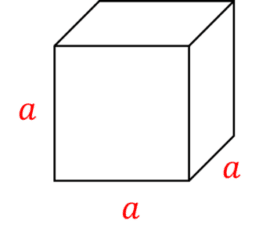The volume of a cube is the length of one side of the cube multiplied by itself three times:

$\text{Volume of cube}=\textcolor{red}{a}\times \textcolor{red}{a}\times \textcolor{red}{a}=\textcolor{red}{a}^{3}$

where $\textcolor{red}{a}$ is the length of one side.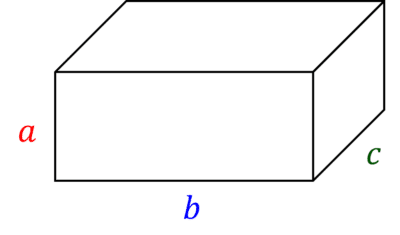The volume of a cuboid is the product of the three different side lengths:

$\text{Volume of cuboid}=\textcolor{red}{a}\times \textcolor{blue}{b}\times \textcolor{green}{c}$

where $\textcolor{red}{a}$, $\textcolor{blue}{b}$ and $\textcolor{green}{c}$ are the three different side lengths of the cuboid.

Level 4-5GCSEKS3AQAEdexcelOCRCambridge iGCSE
Level 4-5GCSEKS3AQAEdexcelOCRCambridge iGCSE

## Volume of Prisms

The formula for the volume of a prism is:

$\text{Volume of prism }=\textcolor{red}{\text{ area of cross section }}\times\textcolor{blue}{\text{ length}}$

This is for any prism, including cuboids & cylinders, and you do have to remember this formula.

So, for Cylinders it would be:

$V = \textcolor{red}{\pi r^2 } \times \textcolor{blue}{\text{ length}}$

Example: Below is a triangular prism. The triangular face has base $6$ cm and perpendicular height $5$ cm.

The prism has length $3.5$ cm.

Work out the volume of the shape.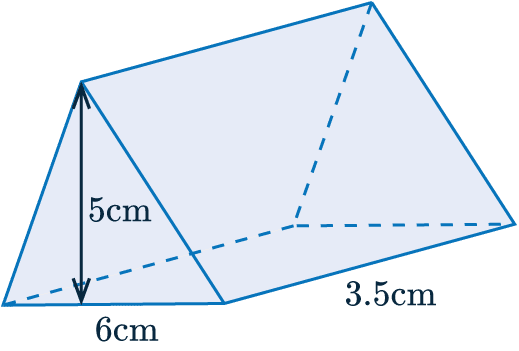In this case, the cross section is a triangle, so we need to multiply the area of the triangle by the length. We get:

$\text{Area of cross section }=\textcolor{red}{\dfrac{1}{2}\times5\times 6}=\textcolor{red}{15}\text{ cm}^2$

Therefore,

$\text{Volume of the prism }=\textcolor{red}{15}\times \textcolor{blue}{3.5}=52.5\text{ cm}^3$.

Level 4-5GCSEKS3AQAEdexcelOCRCambridge iGCSE

## Volume of Pyramids and Cones

The formulas for the volume of pyramids and cones are:

$\text{Volume of pyramid }=\dfrac{1}{3}\times\textcolor{red}{\text{ area of base }}\times\textcolor{blue}{\text{ perpendicular height}}$

$\text{Volume of cone }=\dfrac{1}{3} \times \textcolor{red}{\pi r^2} \times \textcolor{blue}{\text{ perpendicular height}}$

Example: Below is a square-based pyramid.

The base has side-length $14$ mm and the pyramid has perpendicular height $25$ mm.

Work out the volume of the pyramid.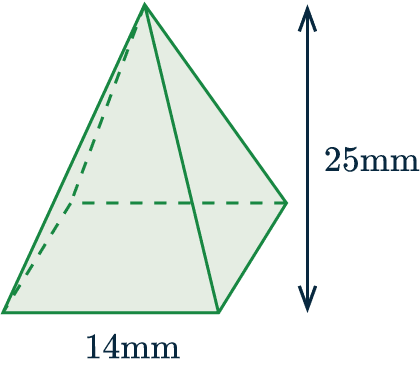For this question we have a square base, so we have to find one third of the area of the square times by the height.

We get:

$\text{Area of base }= \textcolor{red}{14^2}= \textcolor{red}{196}\text{ mm}^2$

Therefore,

$\text{Volume of the pyramid }=\dfrac{1}{3} \times \textcolor{red}{196}\times\textcolor{blue}{25}=1633.3 \text{ mm}^3$

Level 4-5GCSEAQAEdexcelOCRCambridge iGCSE

## Volume of a Sphere

The formula for the volume of a sphere is:

$\text{Volume of a sphere} = \dfrac{4}{3}\textcolor{red}{\pi} \textcolor{blue}{r}^3$

Example: Below is a sphere with radius $\textcolor{blue}{4}$ cm

Calculate the volume of the sphere.

Give your answer to $3$ significant figures.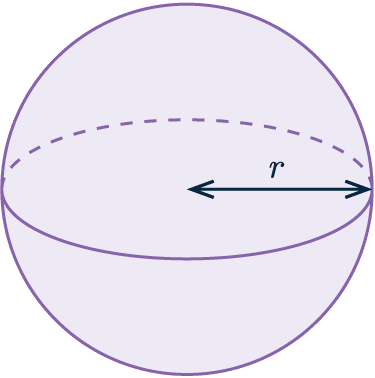We know the radius of the sphere is $4$ cm, so we need to input this into the formula.

Volume of a sphere = $\dfrac{4}{3} \textcolor{red}{\pi} (\textcolor{blue}{4})^3 = 268 \text{ cm} ^3$ ($3$ sf)

Level 4-5GCSEAQAEdexcelOCRCambridge iGCSE

## Example: Composite Shape

The shape below was made by attaching a cone to the top of a cylinder.

The base of the cylinder has radius $4$ mm, the height of the cylinder portion is $3$ mm, and the height of the cone portion is $5.5$ mm.

Calculate the volume of the whole shape.

[4 marks]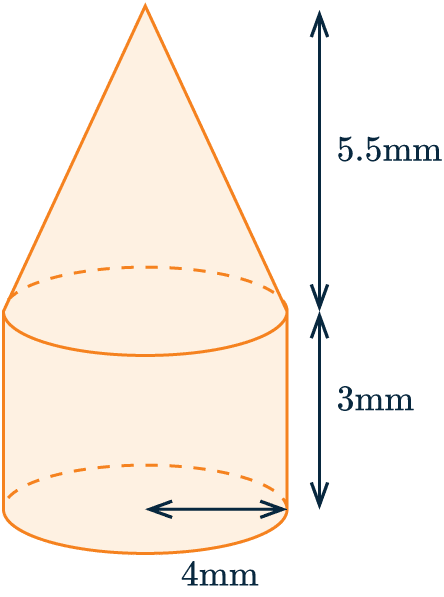So, to work out the volume of the shape, we need to work out the two volumes separately.

Firstly, the cylinder is a type of prism, so we calculate the following:

$\text{volume of cylinder}=\pi\times4^2\times3=48\pi$

Next, we have to work out the volume of the cone:

The vertical height $= 5.5$ mm and the radius $= 4$ mm.

Therefore, we get

$\text{volume of cone }=\dfrac{1}{3}\pi\times4^2\times5.5=\dfrac{88}{3}\pi$

Then, the volume of the shape is the sum of these two answers:

$\text{volume of whole shape }=48\pi+\dfrac{88}{3}\pi$

$= 242.9498...=242.9\text{ mm}^3\text{ (1 dp)}$

Level 4-5GCSEAQAEdexcelOCRCambridge iGCSE

## Volume of 3D Shapes Example Questions

To find the volume we simply have to multiply all three lengths together:

$\text{volume}= 3\times12 \times16=576\text{cm}^3$Gold Standard Education

The volume of a square base pyramid is given by the following formula:

$\text{volume}= \dfrac{1}{3} \times \text{base area} \times \text{height}$

Substituting the values given in the question into the equation above, we find that,

$\text{volume}= \dfrac{1}{3} \times 5^{2} \times 12 =100 \text{m}^3$Gold Standard Education

So, to work out the volume of a prism we must multiply the area of the cross section by the length. In this case, the cross section is a trapezium, and the area of the trapezium is:

$\text{area of cross section }=\dfrac{1}{2}\times(45+60)\times20= 1,050\text{ cm}^2$

The length of the prism is 80 cm, so we get:

$\text{volume of prism }=1,050\times80=84,000\text{ cm}^3$Gold Standard Education

This may seem a little different, but it actually comes up a lot.

First, we must write the volume in terms of $x$. The volume of a pyramid is one third of the area of the base times by the perpendicular height. We know the area of the base is $18\text{cm}^2$, and the expression we’re given for the height is $x+5$, so the volume is

$\dfrac{1}{3}\times18\times(x+5)=6(x+5)$

Now, the question also gave us the volume: $54\text{cm}^3$, so we can equate this value to the expression we found above and voila, we have an equation:

$6(x+5)=54$

Now, we solve this equation to find $x$. First, divide both sides by 6 to get:

$x+5=54\div6=9$

Then, subtracting 5 from both sides we get the answer to be:

$x=9-5=4\text{cm}$Gold Standard Education

We will work out the volume of the cylinder first, then the hemisphere, and add together the values.

A cylinder is a prism which means that to find the volume, we must multiply the area of the circular cross section by its length. The radius of the circle is 2.3m and the length is 5.6m, so we get:

$\text{volume of cylinder }=\pi \times (2.3)^2\times5.6 \approx 93.07 \text{m}^3$

Note: Keep the full answer stored in the calculator for adding it to the other value at the end.

Next, we are given the formula for the volume of a sphere, so to find the volume of the hemisphere we will use this formula and then half the result. The radius of the hemisphere is the same as that of the cylinder, 2.3, so we get:

$\text{volume of hemisphere }=\dfrac{1}{2}\times\left(\dfrac{4}{3}\pi\times(2.3)^3\right)\approx 25.48\text{m}^3$

Therefore, the total volume of the shape is:

$93.0665...+25.4825...=119\text{ m}^3 \text{ (3s.f.)}$Gold Standard Education

## Volume of 3D Shapes Worksheet and Example Questions

### (NEW) Volume of 3D Shapes Exam Style Questions - MME

Level 4-5GCSENewOfficial MME

## Volume of 3D Shapes Drill Questions

Level 1-3GCSE

Level 4-5GCSE

Level 4-5GCSE

Level 4-5GCSE

### Volume of Shapes - Drill Questions

Level 1-3GCSEProduct

£19.99

/month

Learn an entire GCSE course for maths, English and science on the most comprehensive online learning platform. With revision explainer videos & notes, practice questions, topic tests and full mock exams for each topic on every course, it’s easy to Learn and Revise with the MME Learning Portal.

## Related Topics

Level 1-3GCSEKS3

#### Rearranging Formulae

Level 4-5Level 6-7GCSEKS3